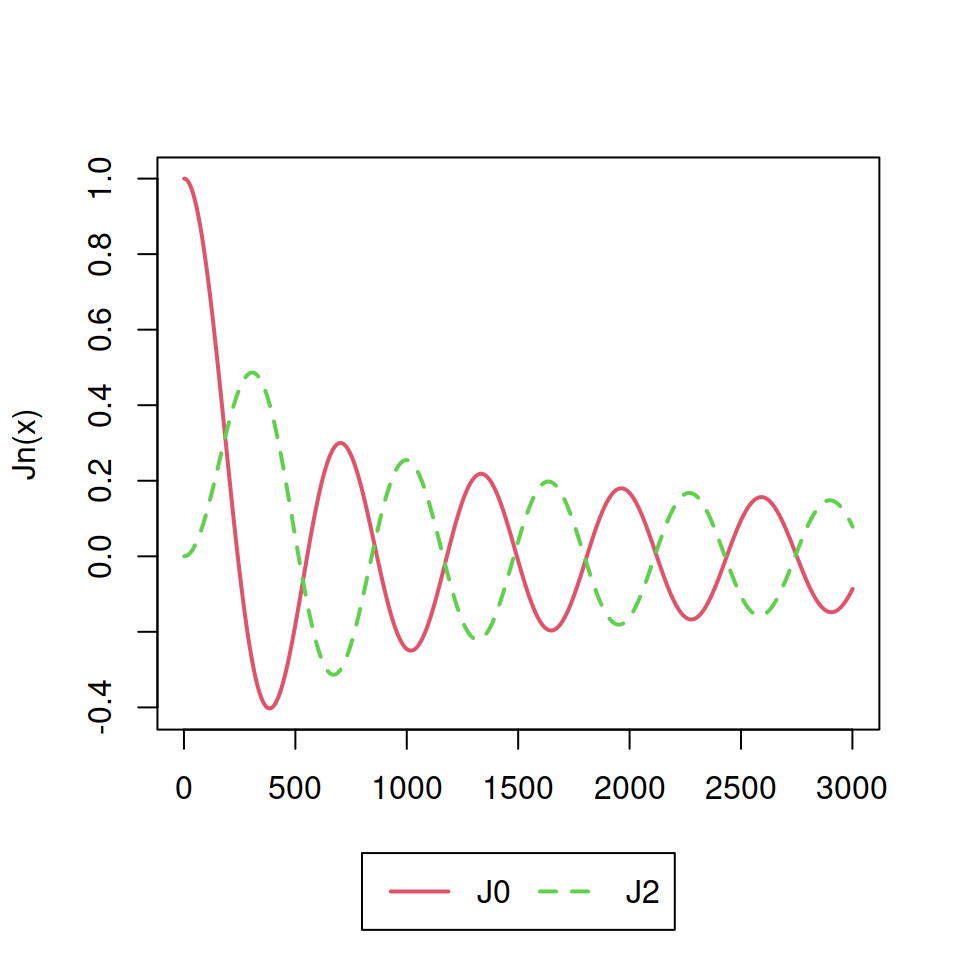# Adding a legend in R

The `legend` function allows adding legends to base R plots.

We are going to use the following plot on the examples of this guide.

``````# Reproducible plot
plotl <- function(points = FALSE, ...) {
x <- seq(0, 30, 0.01)
plot(besselJ(x, 0), col = 2, type = "l",
lwd = 2, ylab = "Jn(x)", xlab = "", ...)
lines(besselJ(x, 2), col = 3,
type = "l", lwd = 2, lty = 2)
if(points == TRUE) {
points(c(1335, 1325),
c(besselJ(x, 0),
besselJ(x, 2)),
pch = c(15, 18), cex = 2, col = 2:3)
}
}

plotl()``````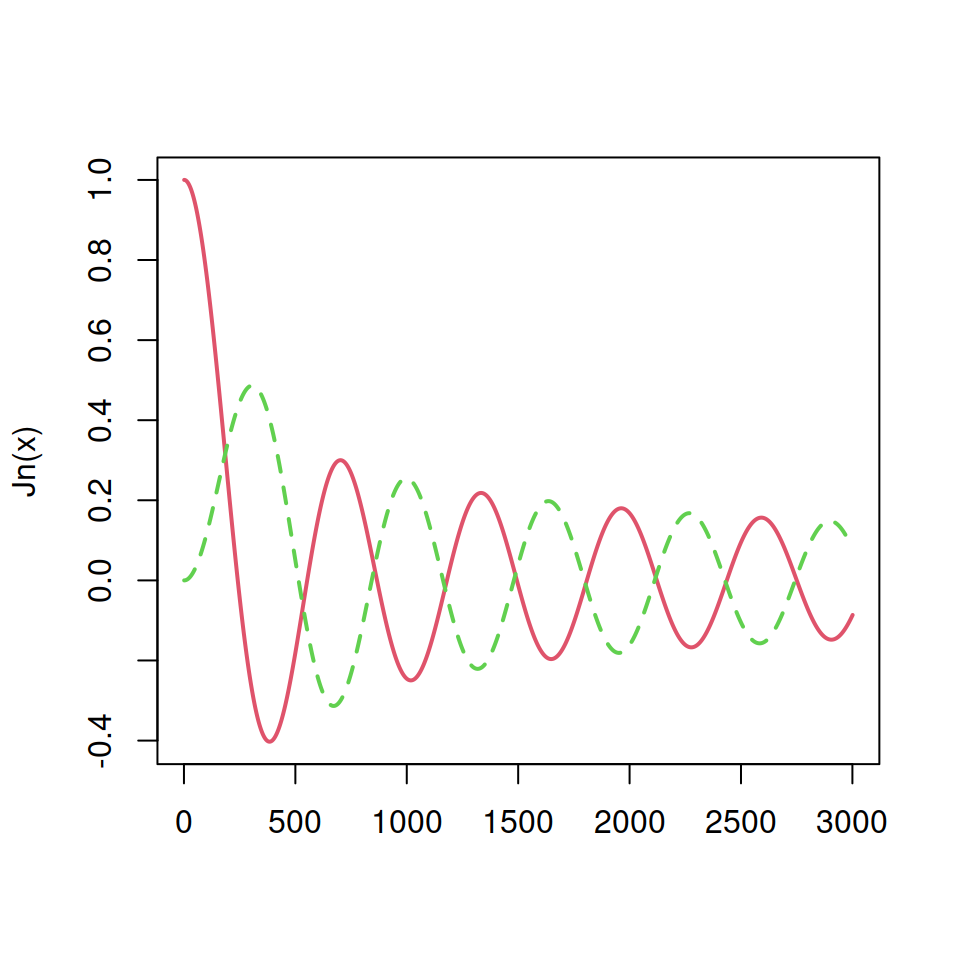## Legend position, lines and fill

Option 1. Set the argument `x` to `"top"`, `"topleft"`, `"topright"`, `"bottom"`, `"bottomleft"`, `"bottomright"`, `"left"`, `"right"` or `"center"`. In this scenario you don’t have to set the argument `y`.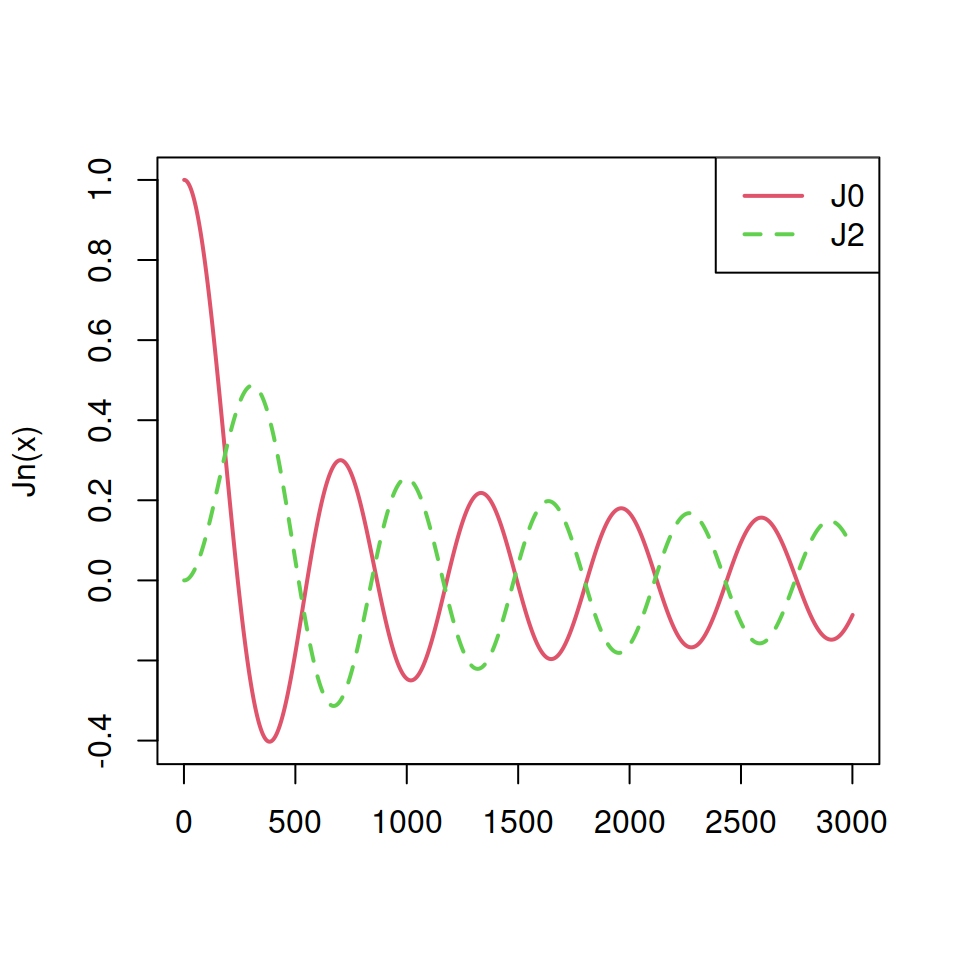The text of the legend can be set with `legend` argument and the line type, width and color with `lty`, `lwd` and `col` arguments, respectively.

``````plotl()
legend(x = "topright",         # Position
legend = c("J0", "J2"), # Legend texts
lty = c(1, 2),          # Line types
col = c(2, 3),          # Line colors
lwd = 2)                # Line widths``````

Option 2. Use the arguments `x` and `y` as coordinates to indicate where to draw the legend.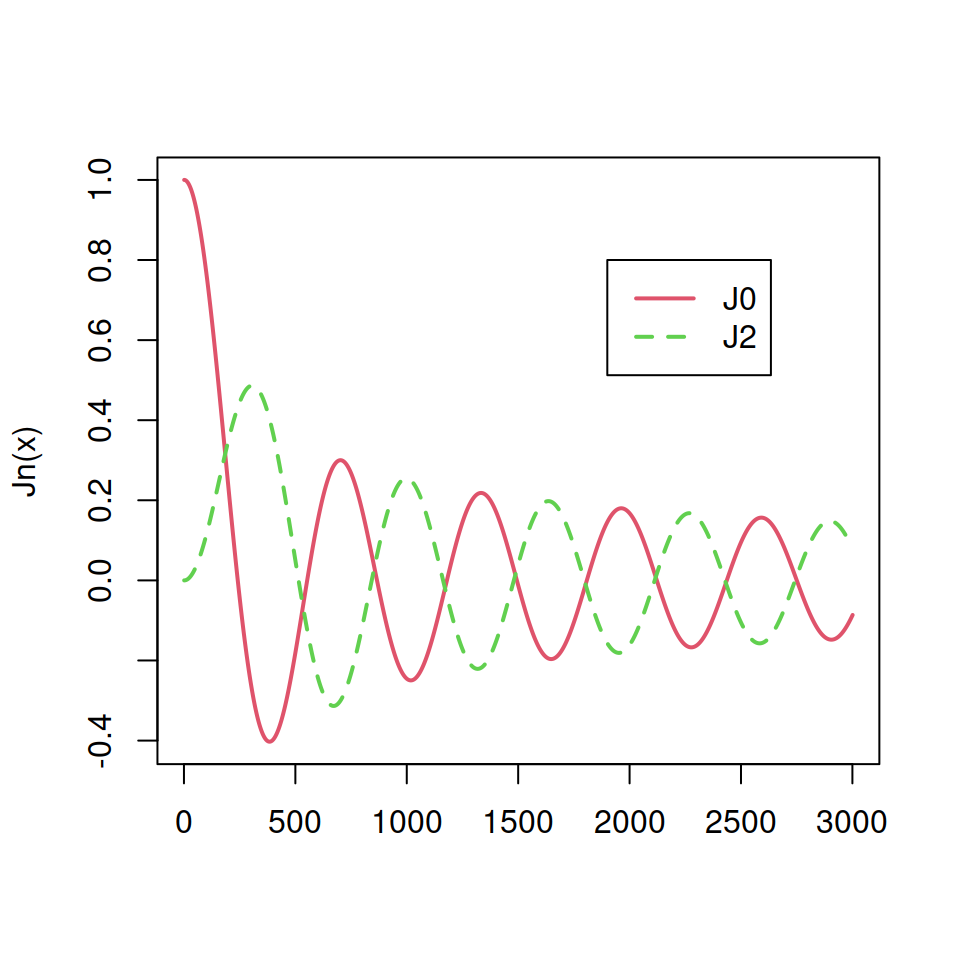``````plotl()
legend(x = 1900, y = 0.8,      # Coordinates
legend = c("J0", "J2"), # Legend texts
lty = c(1, 2),          # Line types
col = c(2, 3),          # Line colors
lwd = 2)                # Line widths``````

Option 3. Use a position and specify a margin from the border with the `inset` argument.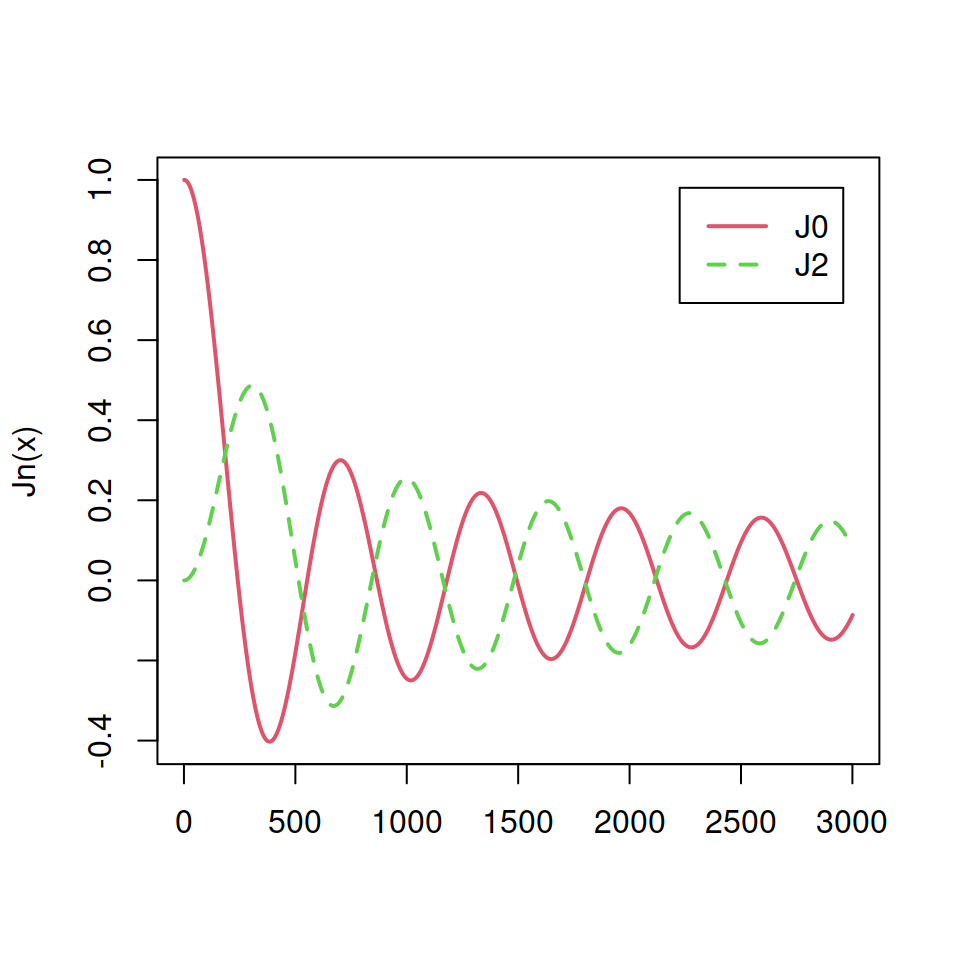The value of the argument represents the distance from the margin as a fraction of the plot region.

``````plotl()
legend("topright",
inset = 0.05, # Distance from the margin
legend = c("J0", "J2"),
lty = c(1, 2),
col = c(2, 3),
lwd = 2)``````

Note that you can also set `pch` symbols over the legend lines if needed.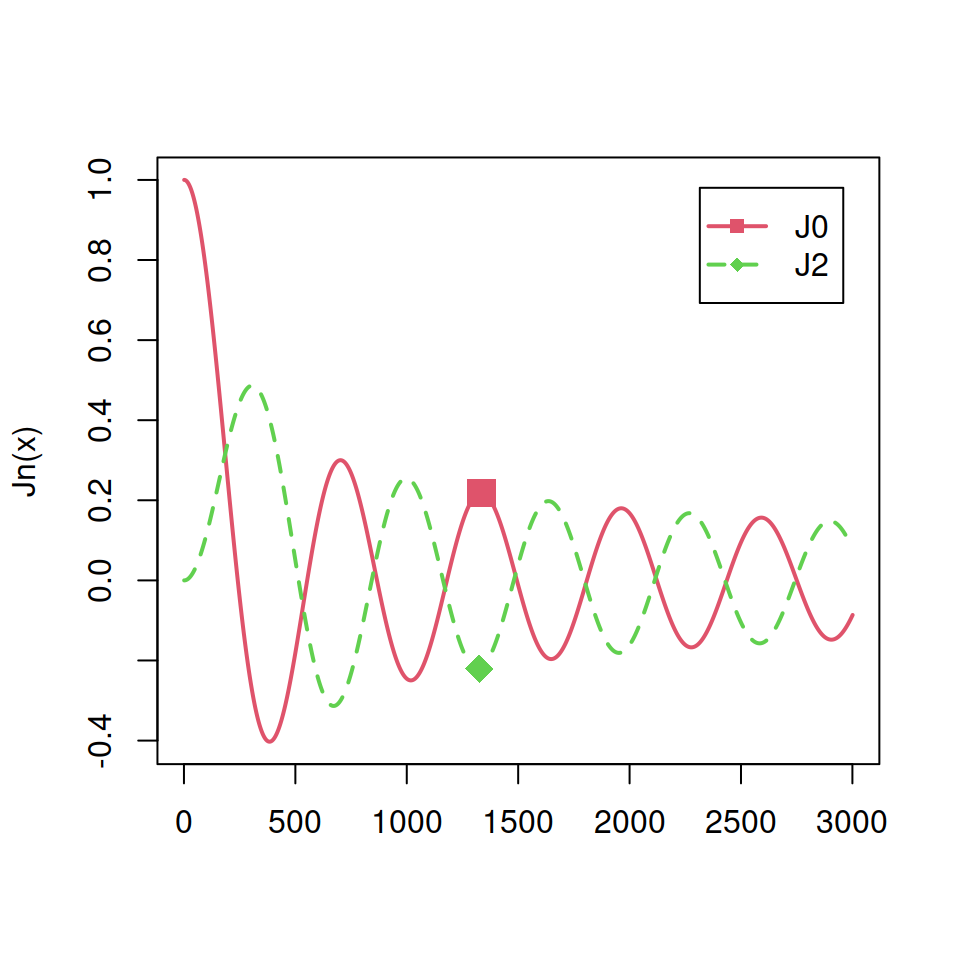``````plotl(points = TRUE)
legend("topright",
inset = 0.05,
legend = c("J0", "J2"),
lty = c(1, 2),
col = c(2, 3),
lwd = 2,
pch = c(15, 18)) # pch symbols``````

If you have a bar plot, pie chart or any other filled plot you can set the argument `fill` instead of `lty`.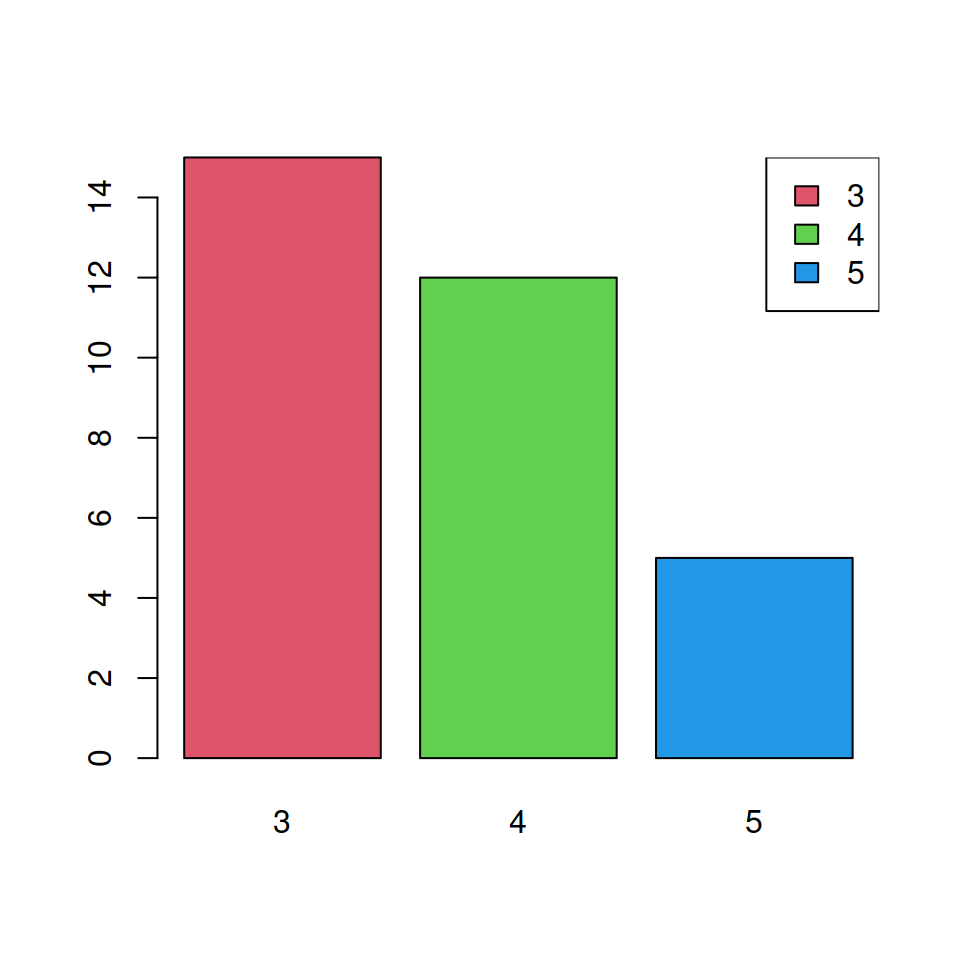You can create filled squares with the `fill` argument, which border color can be modified with the `border` argument.

``````barplot(table(mtcars\$gear), col = 2:4)

legend("topright",
legend = c(3, 4, 5),
fill = 2:4,       # Color of the squares
border = "black") # Color of the border
# of the squares``````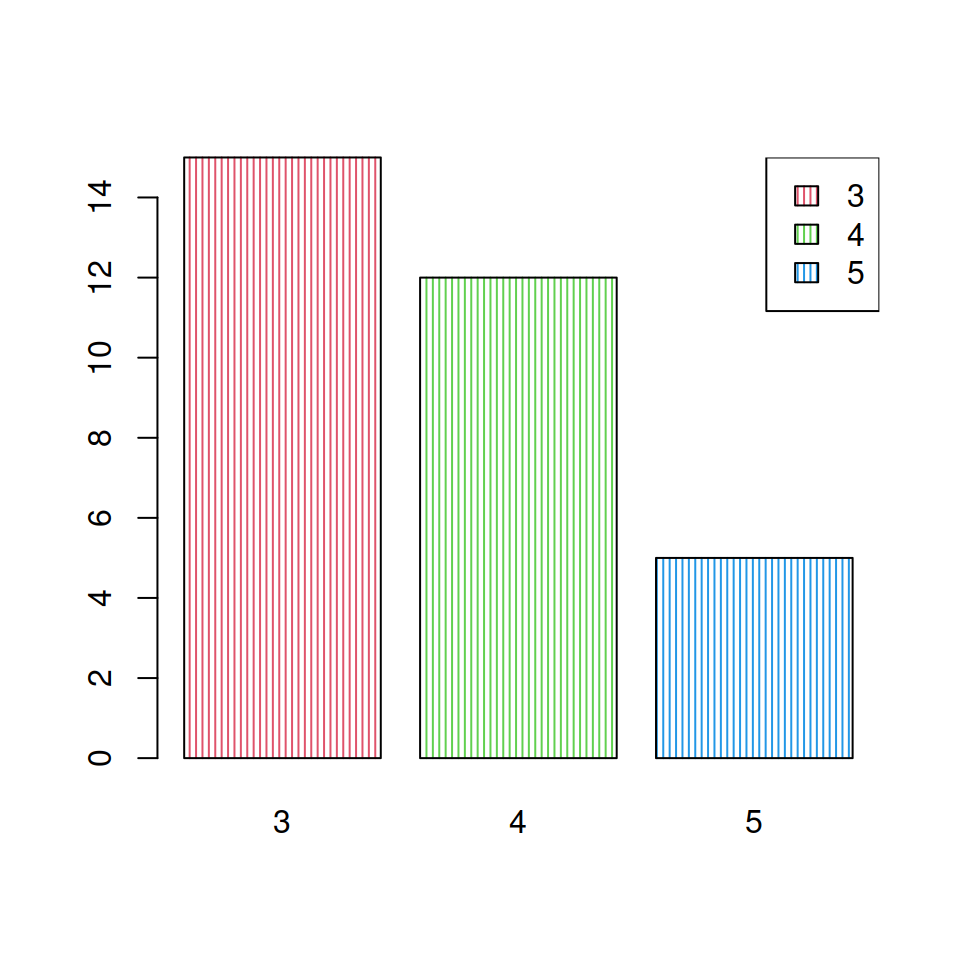If the areas of your plot are shaded you can specify the `density` and `angle` arguments to match them.

``````barplot(table(mtcars\$gear), col = 2:4,
density = 30, angle = 90)

legend("topright",
legend = c(3, 4, 5),
fill = 2:4,
density = 30, # Shading lines density
angle = 90)   # Angle of the shading lines``````

## Legend orientation

Setting `horiz = FALSE` will display the legend in landscape mode.

``````plotl()
legend("top",
legend = c("J0", "J2"),
lty = c(1, 2),
col = c(2, 3),
lwd = 2,
horiz = TRUE)``````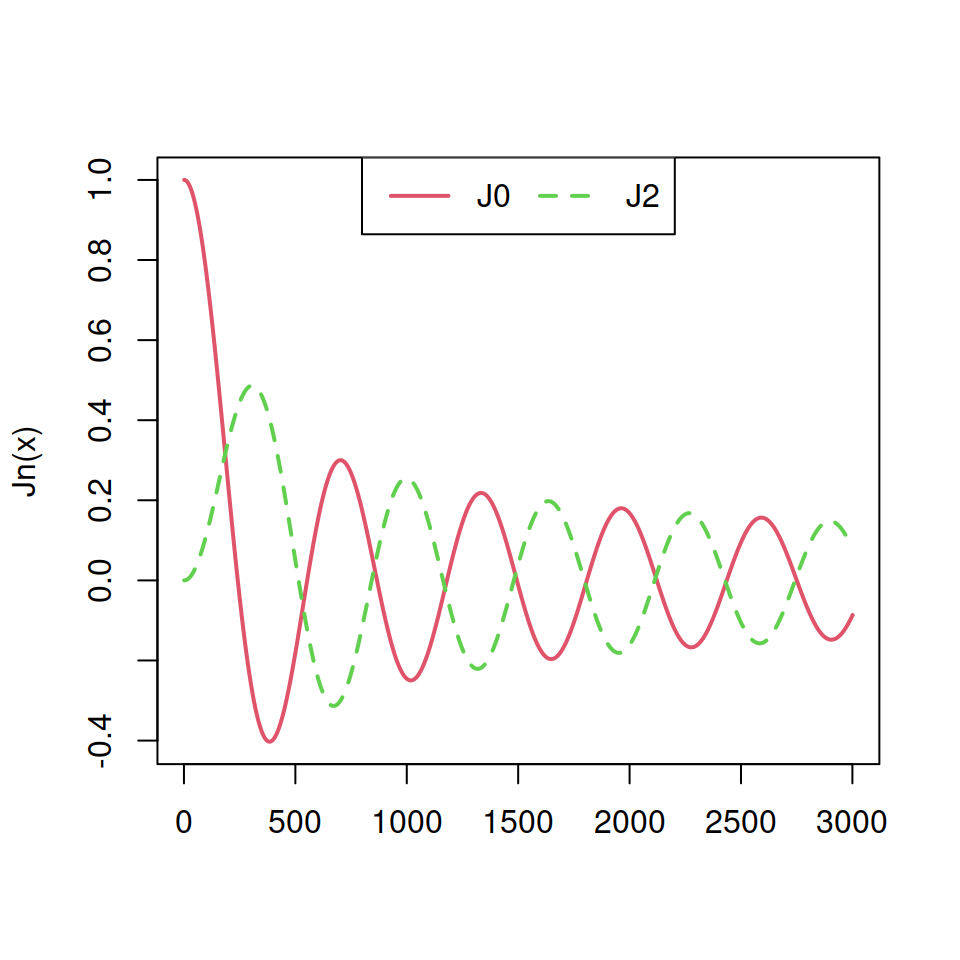You can also specify the number of columns of the legend if `horiz = FALSE` with the argument `ncol`.

``````plotl()
legend("top",
legend = c("J0", "J2"),
lty = c(1, 2),
col = c(2, 3),
lwd = 2,
ncol = 2)``````## Legend title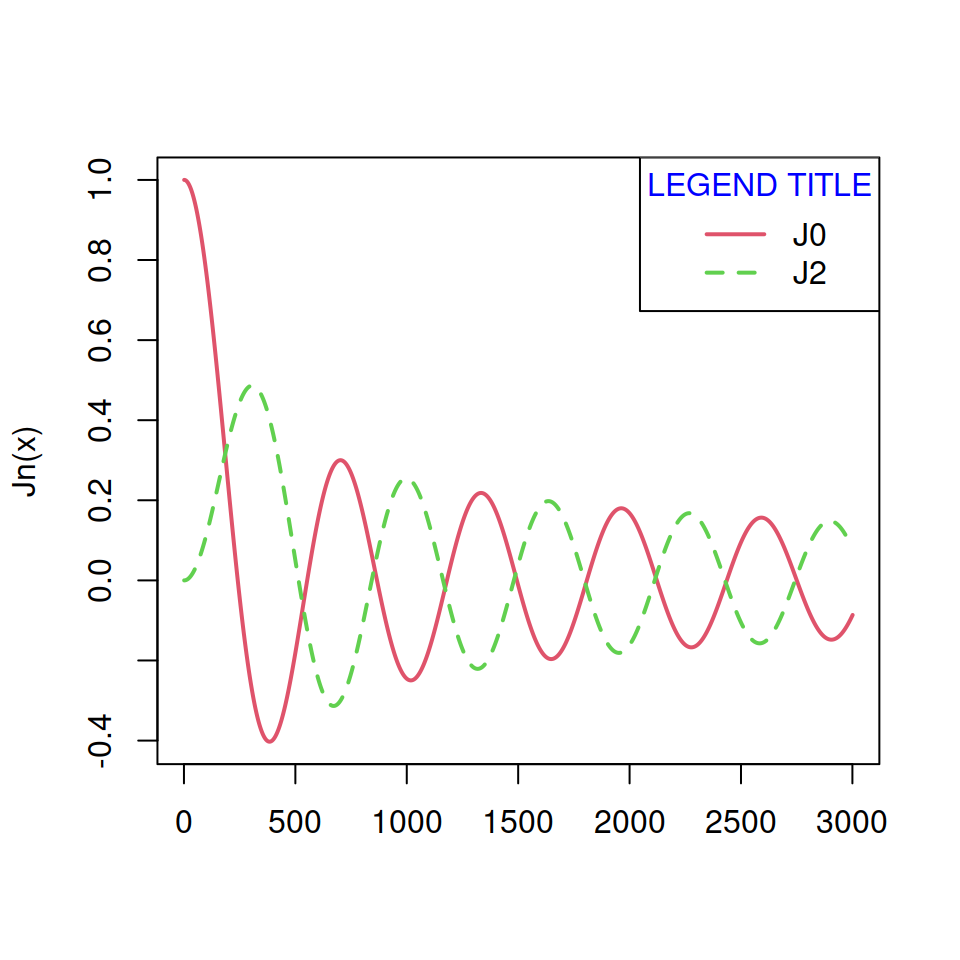It is possible to add a title to a legend and modify its horizontal adjustment and color with the `title`, `title.adj` and `title.col` arguments, respectively.

``````plotl()
legend("topright", legend = c("J0", "J2"),
title = "LEGEND TITLE",  # Title
title.col = "blue", # Color of the title
lty = c(1, 2), col = c(2, 3), lwd = 2)``````

## Box border and background color of the legend

The `box.lty`, `box.lwd` and `box.col` arguments allows modifying the line type, width and color of the legend box, respectively.

``````plotl()
legend(1500, 0.9,
legend = c("J0", "J2"),
box.lty = 2, # Line type of the box
box.lwd = 2, # Box line width
box.col = 4, # Box line color
lty = c(1, 2),
col = c(2, 3),
lwd = 2)``````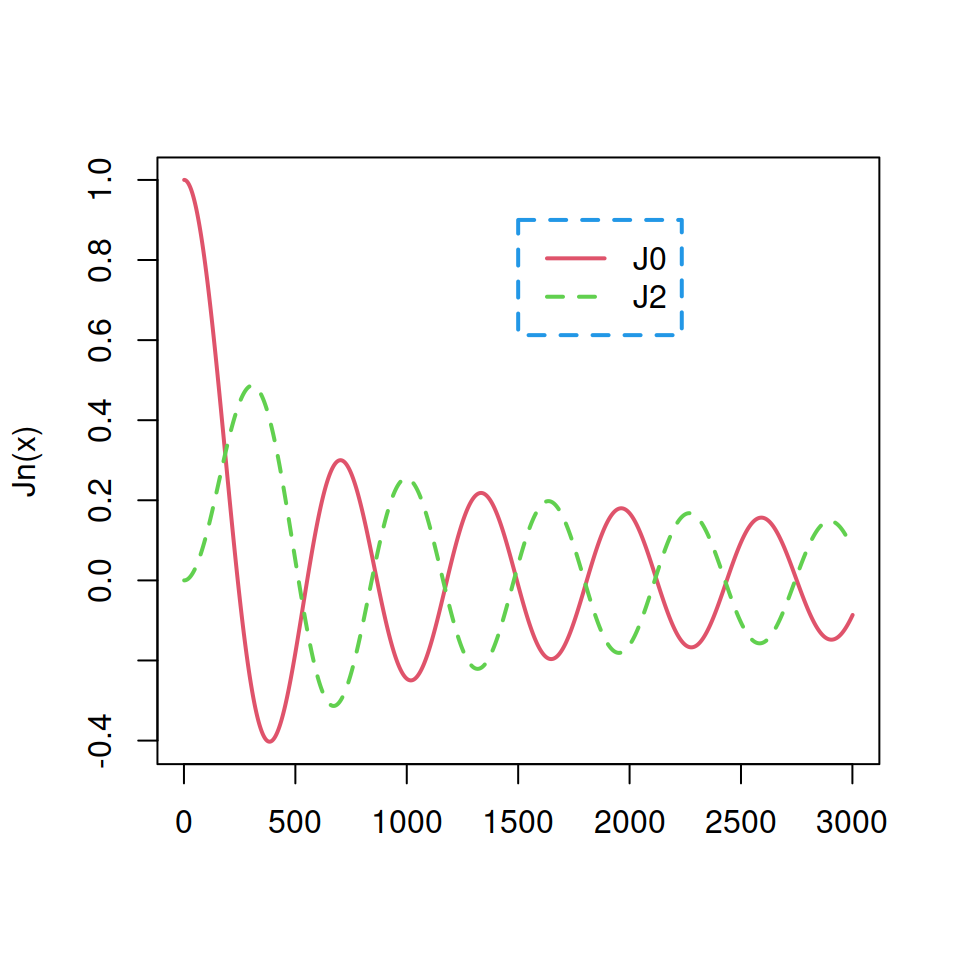In addition to the previous you can also modify the background color of the box with the `bg` argument.

``````plotl()
legend("topright", inset = 0.05,
legend = c("J0", "J2"),
bg = rgb(1, 0, 0, alpha = 0.15),
lty = c(1, 2),
col = c(2, 3),
lwd = 2)``````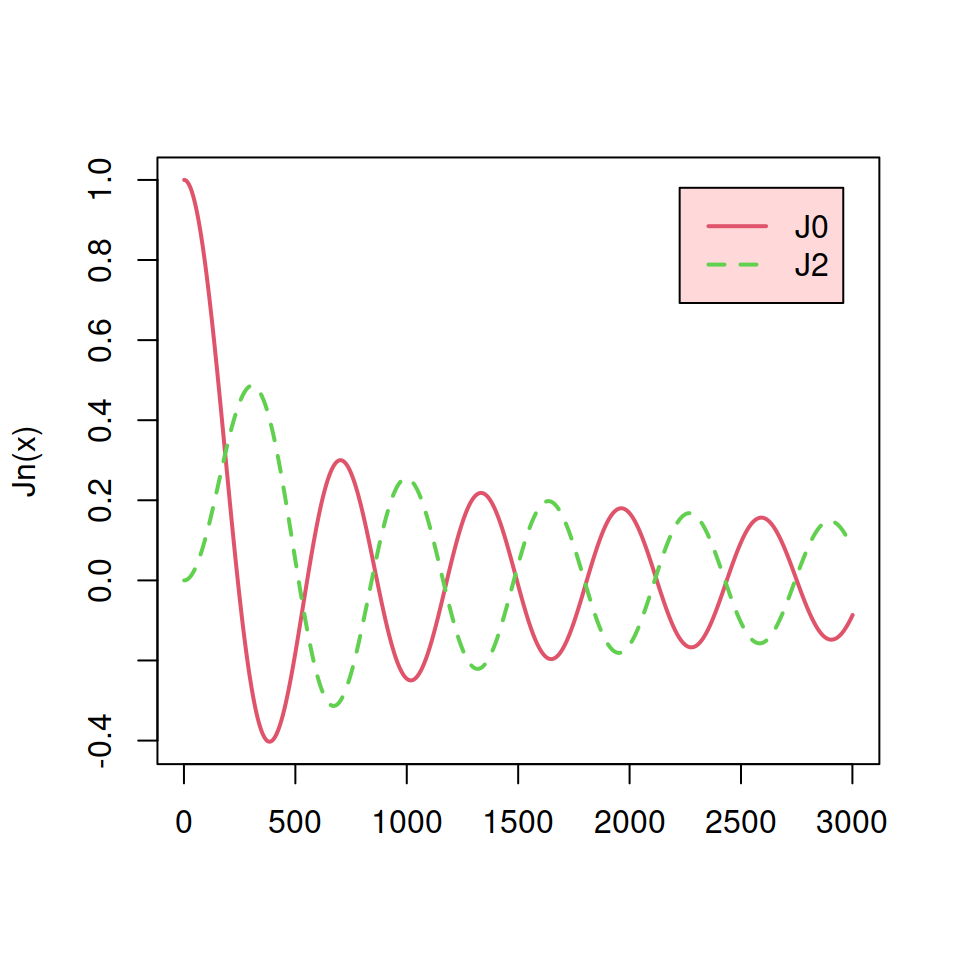## Remove legend box

You can remove the box of the legend by two different methods.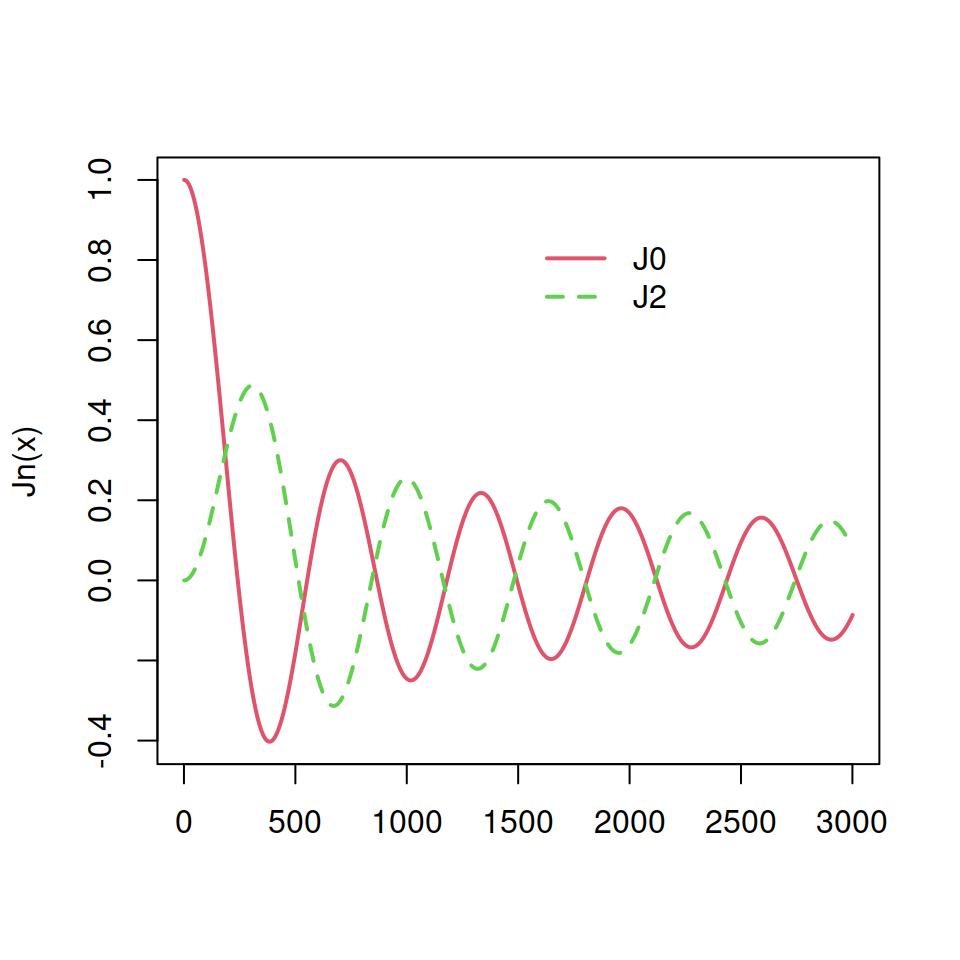Option 1. Set `bty = "n"`.

``````plotl()
legend(1500, 0.9,
legend = c("J0", "J2"),
bty = "n", # Removes the legend box
lty = c(1, 2),
col = c(2, 3),
lwd = 2)``````Option 2. Set `box.lty = 0`

``````plotl()
legend(1500, 0.9,
legend = c("J0", "J2"),
box.lty = 0, # Removes the box line
lty = c(1, 2),
col = c(2, 3),
lwd = 2)``````

## Legend size

The `cex` argument controls the size of the legend. The default value is 1.

``````plotl()
legend("topright",
legend = c("J0", "J2"),
lty = c(1, 2),
col = c(2, 3),
cex = 1.5, # Legend size
lwd = 2)``````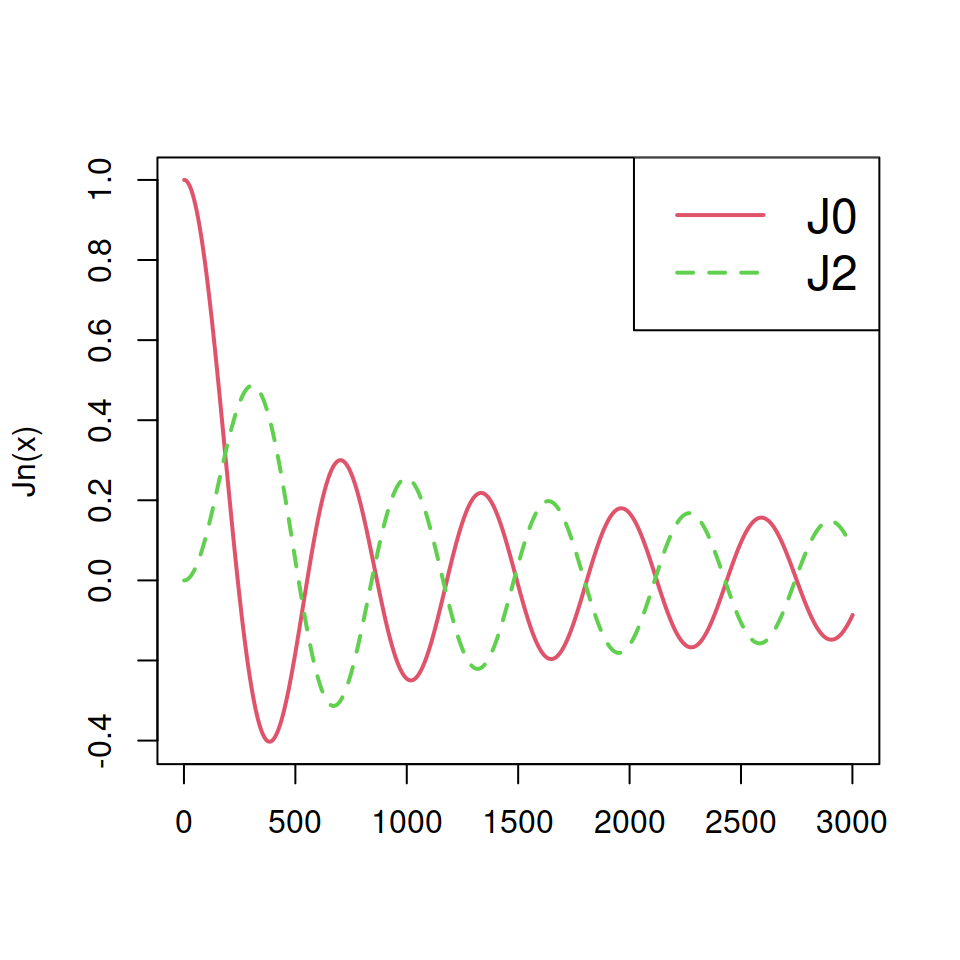## Legend outside the plot

In some scenarios you will need to set the legend outside of the plot because it doesn’t fit inside. For that purpose you will need to increase the margins of the plot, set the legend, use `xpd = TRUE` and fine tune the values of the `inset` argument.

``````# Make the window wider than taller
# windows(width = 5.5, height = 5)

# Save current graphical parameters

# Change the margins of the plot
# (the fourth is the right margin)
par(mar = c(6, 5, 4, 6.5))

plotl()
legend(x = "topright",
inset = c(-0.35, 0), # You will need to fine-tune the first
# value depending on the windows size
legend = c("J0", "J2"),
lty = c(1, 2),
col = c(2, 3),
lwd = 2,
xpd = TRUE) # Needed to put the legend outside the plot

# Back to the default graphical parameters
on.exit(par(opar))``````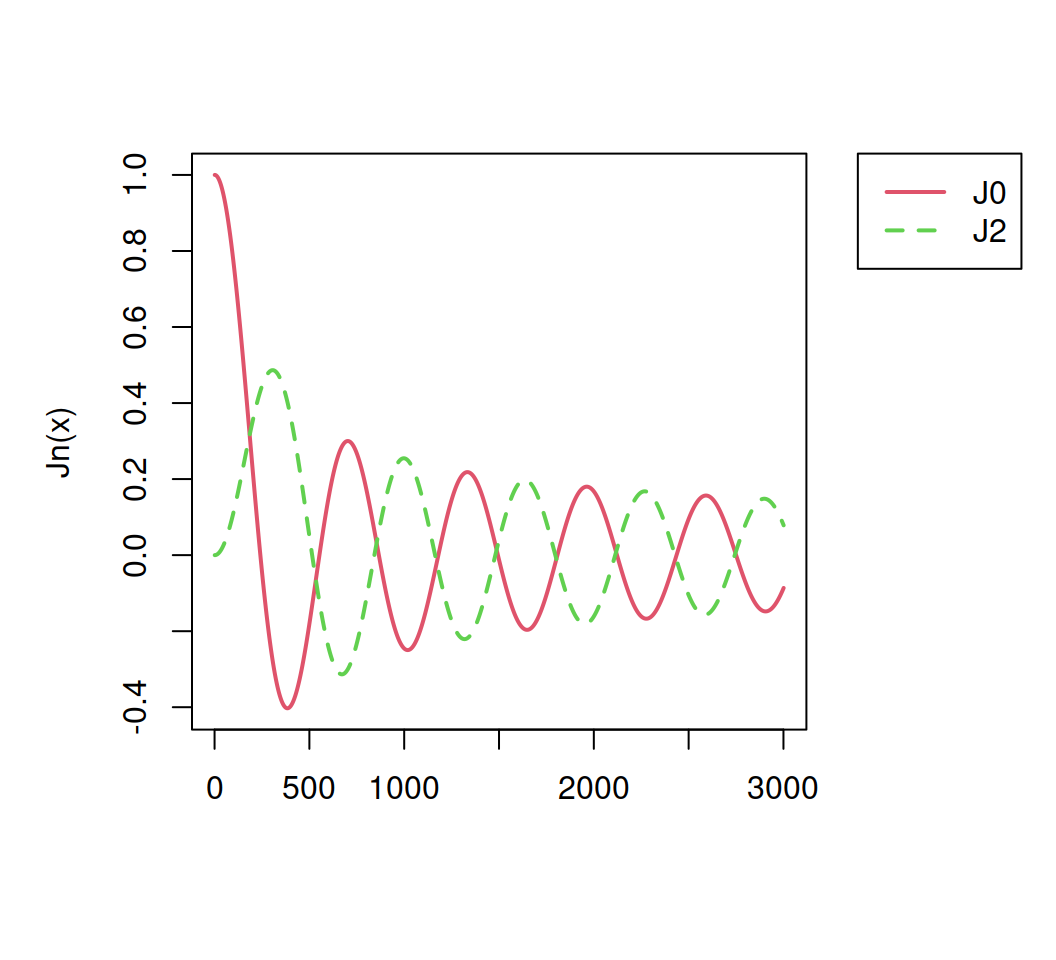### Legend under the plot

An alternative is to set the legend below (or above) the plot following the same approach we followed before but increasing the bottom or upper margins.

``````# windows(width = 5, height = 5)

# Save current graphical parameters

# Change the margins of the plot (the first is the bottom margin)
par(mar = c(6, 4.1, 4.1, 2.1))

plotl()
legend(x = "bottom",
inset = c(0, -0.35), # You will need to fine-tune the second
# value depending on the windows size
legend = c("J0", "J2"),
lty = c(1, 2),
col = c(2, 3),
lwd = 2,
xpd = TRUE, # You need to specify this graphical parameter to add
# the legend outside the plot area
horiz = TRUE) # Horizontal legend

# Back to the default graphical parameters
on.exit(par(opar))``````